# Integral of xln(x)

In this post I am going to explain step-by-step how to integrate the function xln(x). We can solve it by using integration by parts (click here if you want to know more about it), and as you will see something interesting will happen!Graph of the function f(x)=xln(x) in red and the F(x) in blue.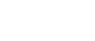Let’s let u=x, so that du=dx. If we let u=ln(x), it means that dv=xdu and so v=x²/2, and we don’t want the degree of the x to increase. To find v, we need to integrate dv, and as we saw here, the integral of ln(x) is x(ln(x)-1); therefore: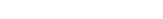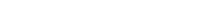Now we can multiply u and v cross-wise and v and du horizontally: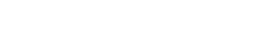Let’s apply linearity: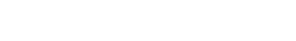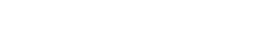At this point you can notice that we have the integral we had at the beginning; this means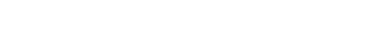We can move the integral on the right-hand side to the left and treat everything like an equation: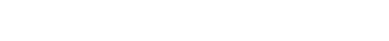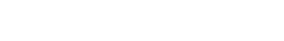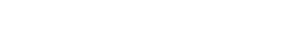And this is the solution to the integral!